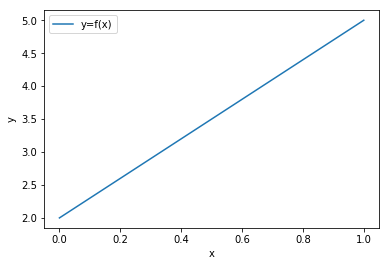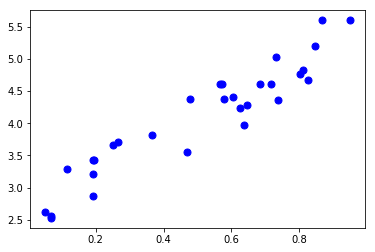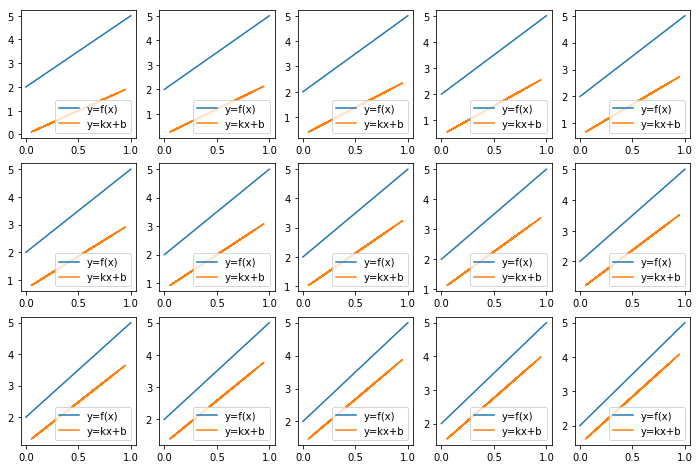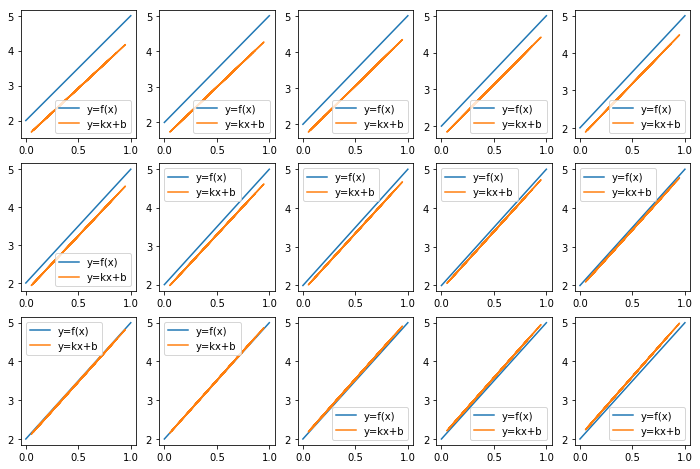Licensed to the Apache Software Foundation (ASF) under one or more contributor license agreements; and to You under the Apache License, Version 2.0.

# Train a linear regression model¶

In this notebook, we are going to use the tensor module from PySINGA to train a linear regression model. We use this example to illustrate the usage of tensor of PySINGA. Please refer the documentation page to for more tensor functions provided by PySINGA.

In :
from __future__ import division
from __future__ import print_function
from builtins import range
from past.utils import old_div

%matplotlib inline
import numpy as np
import matplotlib.pyplot as plt


To import the tensor module of PySINGA, run

In :
from singa import tensor


## The ground-truth¶

Our problem is to find a line that fits a set of 2-d data points. We first plot the ground truth line,

In :
a, b = 3, 2
f = lambda x: a * x + b
gx = np.linspace(0.,1,100)
gy = [f(x) for x in gx]
plt.plot(gx, gy,  label='y=f(x)')
plt.xlabel('x')
plt.ylabel('y')
plt.legend(loc='best')

Out:
<matplotlib.legend.Legend at 0x7fee42985f60>## Generating the trainin data¶

Then we generate the training data points by adding a random error to sampling points from the ground truth line. 30 data points are generated.

In :
nb_points = 30

# generate training data
train_x = np.asarray(np.random.uniform(0., 1., nb_points), np.float32)
train_y = np.asarray(f(train_x) + np.random.rand(30), np.float32)
plt.plot(train_x, train_y, 'bo', ms=7)

Out:
[<matplotlib.lines.Line2D at 0x7fede8b1c588>]## Training via SGD¶

Assuming that we know the training data points are sampled from a line, but we don't know the line slope and intercept. The training is then to learn the slop (k) and intercept (b) by minimizing the error, i.e. ||kx+b-y||^2.

1. we set the initial values of k and b (could be any values).
2. we iteratively update k and b by moving them in the direction of reducing the prediction error, i.e. in the gradient direction. For every iteration, we plot the learned line.
In :
def plot(idx, x, y):
global gx, gy, axes
# print the ground truth line
axes[idx//5, idx%5].plot(gx, gy, label='y=f(x)')
# print the learned line
axes[idx//5, idx%5].plot(x, y, label='y=kx+b')
axes[idx//5, idx%5].legend(loc='best')

# set hyper-parameters
max_iter = 15
alpha = 0.05

# init parameters
k, b = 2.,0.


SINGA tensor module supports basic linear algebra operations, like + - * /, and advanced functions including axpy, gemm, gemv, and random function (e.g., Gaussian and Uniform).

SINGA Tensor instances could be created via tensor.Tensor() by specifying the shape, and optionally the device and data type. Note that every Tensor instance should be initialized (e.g., via set_value() or random functions) before reading data from it. You can also create Tensor instances from numpy arrays,

• numpy array could be converted into SINGA tensor via tensor.from_numpy(np_ary)
• SINGA tensor could be converted into numpy array via tensor.to_numpy(); Note that the tensor should be on the host device. tensor instances could be transferred from other devices to host device via to_host()

Users cannot read a single cell of the Tensor instance. To read a single cell, users need to convert the Tesnor into a numpy array.

In :
# to plot the intermediate results
fig, axes = plt.subplots(3, 5, figsize=(12, 8))
x = tensor.from_numpy(train_x)
y = tensor.from_numpy(train_y)
# sgd
for idx in range(max_iter):
y_ = x * k + b
err = y_ - y
loss = old_div(tensor.sum(err * err), nb_points)
print('loss at iter %d = %f' % (idx, loss))
da1 = old_div(tensor.sum(err * x), nb_points)
db1 = old_div(tensor.sum(err), nb_points)
# update the parameters
k -= da1 * alpha
b -= db1 * alpha
plot(idx, tensor.to_numpy(x), tensor.to_numpy(y_))

loss at iter 0 = 9.660777
loss at iter 1 = 8.484187
loss at iter 2 = 7.452227
loss at iter 3 = 6.547118
loss at iter 4 = 5.753264
loss at iter 5 = 5.056990
loss at iter 6 = 4.446301
loss at iter 7 = 3.910676
loss at iter 8 = 3.440886
loss at iter 9 = 3.028838
loss at iter 10 = 2.667435
loss at iter 11 = 2.350451
loss at iter 12 = 2.072425
loss at iter 13 = 1.828568
loss at iter 14 = 1.614680We can see that the learned line is becoming closer to the ground truth line (in blue color).

## Next: MLP example¶

In :
# to plot the intermediate results
fig, axes = plt.subplots(3, 5, figsize=(12, 8))
x = tensor.from_numpy(train_x)
y = tensor.from_numpy(train_y)
# sgd
for idx in range(max_iter):
y_ = x * k + b
err = y_ - y
loss = old_div(tensor.sum(err * err), nb_points)
print('loss at iter %d = %f' % (idx, loss))
da1 = old_div(tensor.sum(err * x), nb_points)
db1 = old_div(tensor.sum(err), nb_points)
# update the parameters
k -= da1 * alpha
b -= db1 * alpha
plot(idx, tensor.to_numpy(x), tensor.to_numpy(y_))

loss at iter 0 = 1.427076
loss at iter 1 = 1.262527
loss at iter 2 = 1.118196
loss at iter 3 = 0.991600
loss at iter 4 = 0.880558
loss at iter 5 = 0.783158
loss at iter 6 = 0.697723
loss at iter 7 = 0.622783
loss at iter 8 = 0.557046
loss at iter 9 = 0.499382
loss at iter 10 = 0.448799
loss at iter 11 = 0.404426
loss at iter 12 = 0.365499
loss at iter 13 = 0.331350
loss at iter 14 = 0.301391In [ ]: